# Maximum call stack size exceeded Error in TypeScript [Fixed]Last updated: Jan 23, 2023
3 min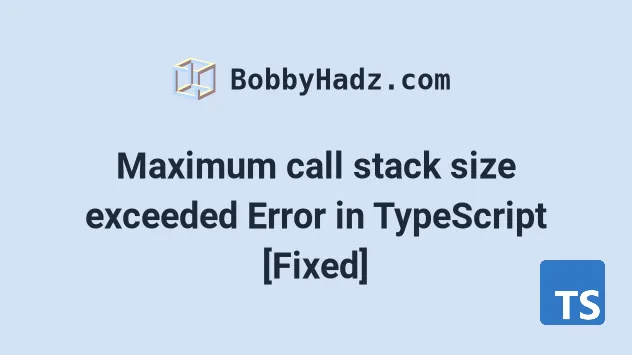## #Maximum call stack size exceeded Error in TypeScript

The "RangeError: Maximum call stack size exceeded" occurs when a function is being called so many times that the invocations exceed the call stack limit.

To solve the error, track down the cause or specify a base case that has to be met to exit the recursion.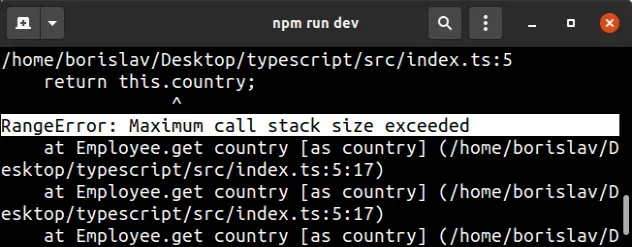## #How the error occurs with a getter method

Here is an example of how the error occurs.

index.ts
```Copied!```class Employee {
protected _country = 'Germany';

get country(): string {
// ⛔️ Error: RangeError: Maximum call stack size exceeded
return this.country; // 👈️ should be this._country
}
}

const emp = new Employee();

console.log(emp.country);
``````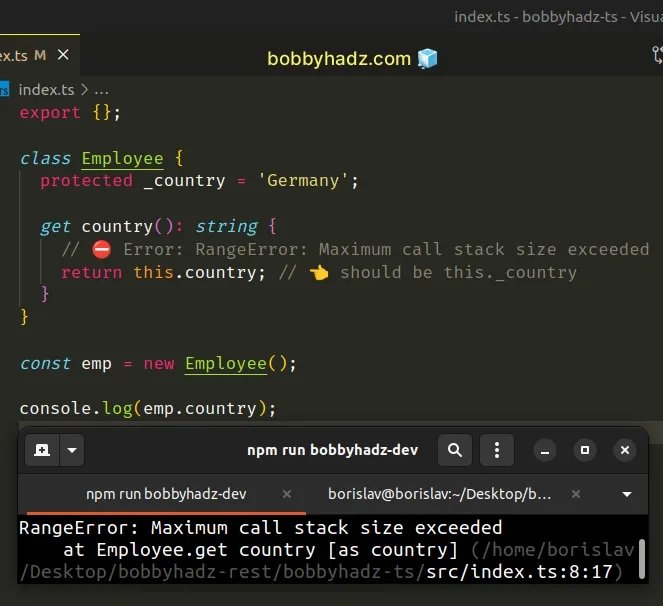The problem is that the `country` getter function keeps calling itself until the invocations exceed the call stack limit.

## #How the error occurs with a regular function

Here is another example of how the error occurs.

index.ts
```Copied!```function example() {
example();
}

// ⛔️ Error: RangeError: Maximum call stack size exceeded
example();
``````

The most common cause of the error is having a function that keeps calling itself.

## #Solve the error when using getter methods

To solve the error when using getter methods, we have to access the property by prefixing it with an underscore instead of calling the getter.

index.ts
```Copied!```class Employee {
protected _country = 'Germany';

get country(): string {
return this._country;
}
}
``````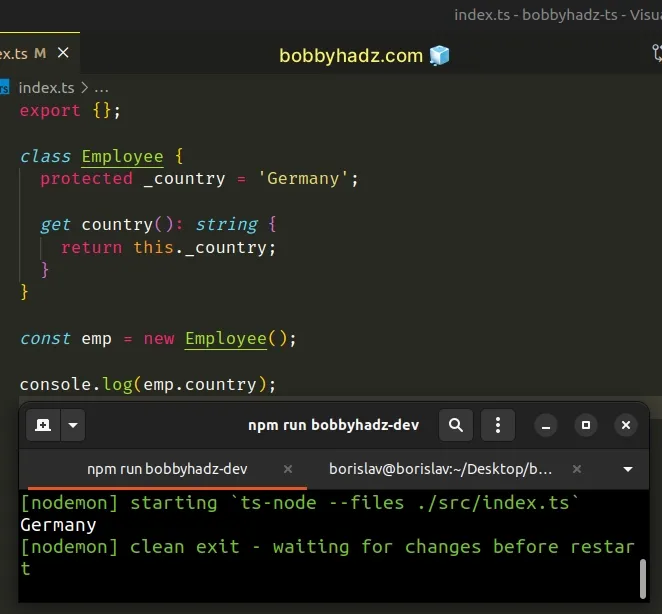## #Solve the error when working with regular functions

To solve the error when working with functions, we have to specify a condition at which the function stops calling itself.

index.ts
```Copied!```let counter = 0;

function example(num: number) {
if (num < 0) {
return;
}

counter += 1;
example(num - 1);
}

example(3);

console.log(counter); // 👉️ 4
``````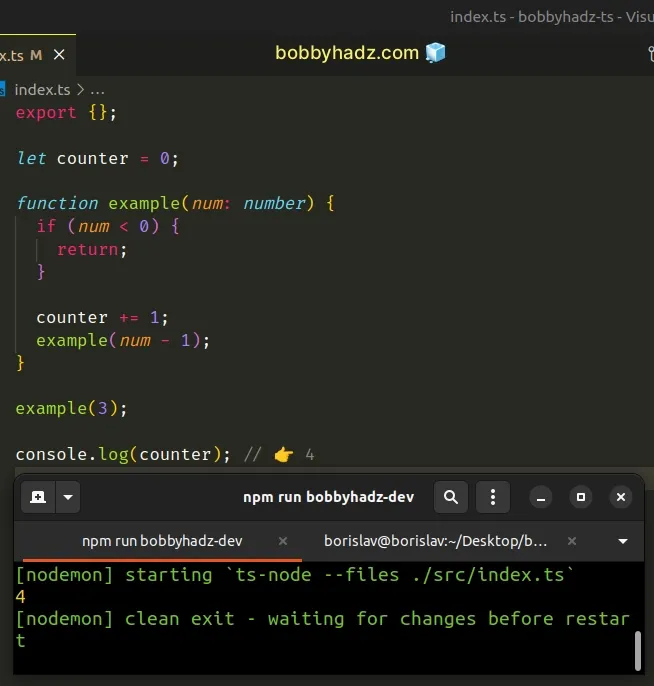This time we check if the function was invoked with a `number` that is less than `0` on every invocation.

If the number is less than `0`, we simply return from the function so we don't exceed the call stack limit.

If the passed-in value is not less than zero, we call the function with the passed in value minus `1`.

This keeps us moving toward the case where the `if` condition is satisfied.

A recursive function calls itself until a condition is met. If there is no condition to be met in your function, it will call itself until the maximum call stack size is exceeded.

## #Make sure you don't have infinite loops

You might also get this error if you have an infinite loop that calls a function somewhere.

index.ts
```Copied!```function sum(a: number, b: number) {
return a + b;
}

while (true) {
sum(10, 10);
}
``````
Our `while` loop keeps calling the function and since we don't have a condition that would exit the loop we eventually exceed the call stack limit.

This works in a very similar way to a function calling itself without a base condition. The same would be the case if you were using a `for` loop.

Here's an example of how to specify a condition that has to be met to exit the loop.

index.ts
```Copied!```function sum(a: number, b: number) {
return a + b;
}

let total = 0;

for (let i = 10; i > 0; i--) {
total += sum(5, 5);
}

console.log(total); // 👉️ 100
``````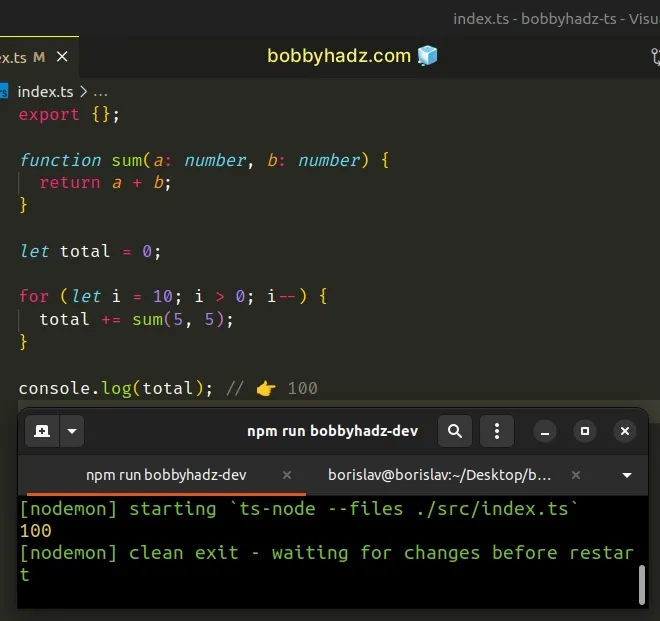If the `i` variable is equal to or less than `0`, the condition in the `for` loop is not satisfied, so we exit the loop.

If you can't track exactly where your error occurs, look at the error message in your browser's console or your terminal (if using Node.js).The screenshot above shows that the error occurred on line `5` in the `index.ts` file.

## #Make sure you don't load the same script multiple times

The error might also occur if you import the same file more than once, e.g. loading the jQuery script multiple times on the same page.

1. Opening the developer tools in your browser by pressing `F12`.
2. Selecting the `Network` tab in your browser's developer tools.